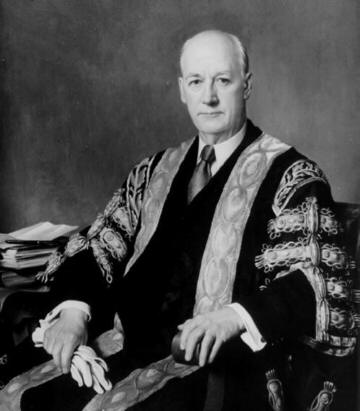# Samuel Beatty (1881-1970) mathematician

 In 1926, Sam Beatty published a problem in the American Mathematical Monthly, and soon thereafter many people were talking about Beatty sequences. By 1995, these sequences had been mentioned in hundreds of mathematical articles and books. What are the attractive sequences? Suppose R is an irrational number greater than 1, and let S be the number satisfying the equation 1/R + 1/S = 1. Let [x] denote the floor function of x, i.e., the greatest integer less than or equal to x. The sequences [nR] and [nS], where n ranges through the set N of positive integers, are the Beatty sequences determined by R. Here's what's so good about them: they partition N; i.e., every positive integer occurs exactly once in one sequence or the other. For example, when R is the golden mean (about 1.618), the two sequences begin with 1, 3, 4, 6, 8, 9, 11, 12, 14, 16, 17, 19, 21 and 2, 5, 7, 10, 13, 15, 18, 20, 23, 26, 28, 31, 34.Sam Beatty was the first person to receive a Ph.D. in mathematics from a Canadian university. He was a colorful teacher and problemist who became the chairman of the mathematics department, and later, the 21st Chancellor, of the University of Toronto.

A long list of references to Beatty sequences and related matters appears in

Kenneth B. Stolarsky "Beatty sequences, continued fractions, and certain shift operators," Canadian Math. Bulletin 19 (1976) 473-482.